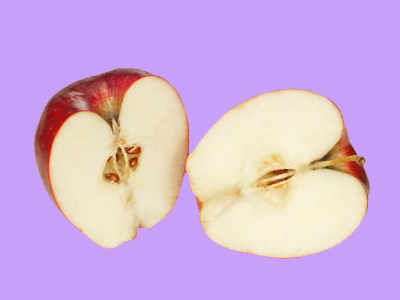If something is halved it is divided into two equally sized parts.

# Year 1 Fractions - Halves

This quiz addresses the requirements of the National Curriculum KS1 Maths and Numeracy for children aged 5 and 6 in year 1. Specifically this quiz is aimed at the section dealing with recognising, finding and naming a half as one of two equal parts of an object, shape or quantity. It gently introduces them to fractions.

Halving numbers or groups of objects is often young children’s first experience of fractions. They may relate this to cutting one object in two, dividing by two or sharing one group into two equal parts. Children should recognise that for halving to be accurate, both parts should be equal in size or amount.

Question 1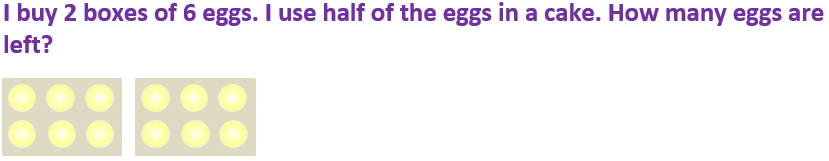2
16
6
4
Using half of the eggs would be the same as using one box only
Question 2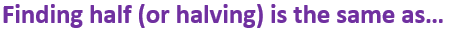Subtracting 2
Multiplying by 2
Dividing by 2
Halving means sharing into 2 equal groups or parts
Question 3Cut it into 4 slices
Cut it into 2 equal parts
Share it with some friends
Eat some quickly
The two halves should be exactly the same size
Question 4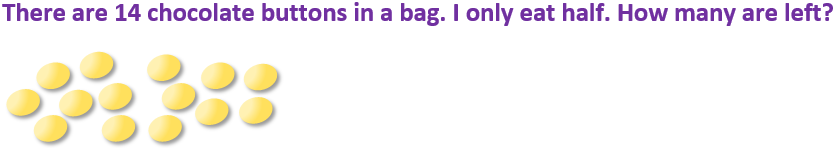7
6
5
10
Half of 14 is 7
Question 516
14
22
10
Sharing 20 into two equal groups would give 10 in each group
Question 6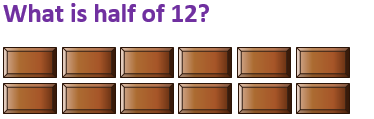6
12
14
24
12 ÷ 2 = 6, so half of 12 is 6
Question 7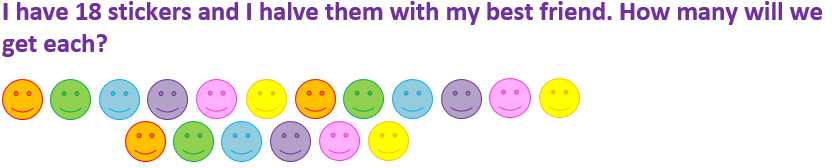14
10
16
9
Half of 10 is 5, half of 8 is 4. 5 + 4 = 9
Question 8Cut some out
Share them into two equal groups
Put them into 4 piles
Put them in a bag
Each group should have exactly the same number of stars
Question 9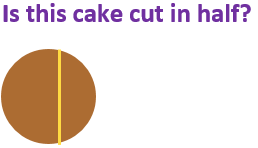Yes, there is a line down the middle
Yes, it has been shared between two people
Yes, there are two pieces
No, the two pieces aren’t the same size
Halves of a shape must be identical
Question 10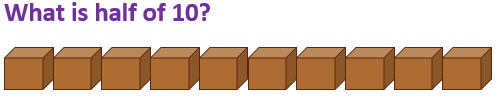8
5
20
35
10 shared into 2 equal groups gives 5 in each group, so half of 10 is 5